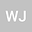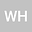Existence of multiple positive solutions for singular p-q-Laplacian problems with critical nonlinearities
••Wei Han
North University of China - South Campus
Author Profile## Abstract

In this article, we consider the following p-q-Laplacian system with singular and critical nonlinearity \begin{equation*} \left \{ \begin{array}{lllll} -\Delta_{p}u-\Delta_{q}u=\frac{h_{1}(x)}{u^{r}}+\lambda\frac{\alpha}{\alpha+\beta}u^{\alpha-1}v^{\beta} \ \ in\ \Omega ,\\ -\Delta_{p}v-\Delta_{q}v=\frac{h_{2}(x)}{v^{r}}+\lambda\frac{\beta}{\alpha+\beta}u^{\alpha}v^{\beta-1} \ \ in\ \Omega, \\ u,v>0 \ \ \ \ \ \ in \ \Omega, \ \ \ \ \ u=v=0 \ \ \ \ \ \ \ on \ \partial\Omega, \end{array} \right. \end{equation*} where Ω is a bounded domain in $\mathbb {R}^{n}$ with smooth boundary $\partial\Omega$. $11,\lambda\in(0,\Lambda_{*})$ is parameter with $\Lambda _{*}$ is a positive constant and $h_{1}(x),h_{2}(x)\in L^{\infty},h_{1}(x),h_{2}(x)>0$. We show the existence and multiplicity of weak solution of equation above for suitable range of $\lambda$.

#### Peer review status:UNDER REVIEW

06 Apr 2021Submitted to Mathematical Methods in the Applied Sciences
08 Apr 2021Assigned to Editor
08 Apr 2021Submission Checks Completed
19 Apr 2021Reviewer(s) Assigned